Courses

# Quantitative Aptitude - Test 2

## 25 Questions MCQ Test SSC CGL Tier 1 Mock Test Series | Quantitative Aptitude - Test 2

Description
This mock test of Quantitative Aptitude - Test 2 for SSC helps you for every SSC entrance exam. This contains 25 Multiple Choice Questions for SSC Quantitative Aptitude - Test 2 (mcq) to study with solutions a complete question bank. The solved questions answers in this Quantitative Aptitude - Test 2 quiz give you a good mix of easy questions and tough questions. SSC students definitely take this Quantitative Aptitude - Test 2 exercise for a better result in the exam. You can find other Quantitative Aptitude - Test 2 extra questions, long questions & short questions for SSC on EduRev as well by searching above.
QUESTION: 1

### Find the approximate area of a trapezium whose parallel sides are 22 cm, 12 cm and the other sides are 8 cm and 6 cm.

Solution: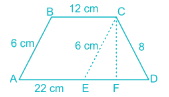Consider the trapezium ABCD,
where AB = 6 cm, BC = 12 cm, CD = 8 cm and AD = 22 cm.
Draw a line from C parallel to AB at E such that ABCE is a parallelogram.
∴ CE = AB = 6 cm and AE = BC = 12 cm.
⇒ ED = AD – AE
⇒ ED = 22 – 12 = 10 cm.
Area of Trapezium ABCD = Area of Parallelogram ABCE + Area of ΔCED
Draw a line through C to ED such that CF is perpendicular to ED.
Area of ΔCED:
From Heron’s formulae,
Δ CED = √[(s) (s - a) (s - b) (s - c)], where s = (a + b + c)/2 and a, b, c are side of the triangle.
In this case, a = 6; b = 8 and c = 10
⇒ s = 24/2 = 12
Area of ΔCED = √(12 × 6 × 4 × 2)
⇒ Area of ΔCED = √(576) = 24 sq. cm.
Area of ΔCED = (1/2) × ED × CF
⇒ 24 = (1/2) × 10 × CF
⇒ CF = 4.8 cm.
Area of Parallelogram ABCE = AE × CF
⇒ Area of Parallelogram ABCE = 12 × 4.8 = 57.6 sq. cm.
Area of trapezium ABCD = Area of parallelogram ABCE + Area of ΔCED
⇒ Area of trapezium ABCD = 57.6 + 24 = 81.6 sq. cm.
∴ Approximate area of the trapezium = 81.6 sq. cm.

QUESTION: 2

### A, B and C enter into a partnership, investing Rs. 6000. A invests Rs. 1000 and B and C invests in the ratio of 2 : 3. Find the profit of C, when the annual profit is Rs. 2400

Solution:

From given data,
Total investment = Rs. 6000
A investment = Rs. 1000
⇒ B and C’s investment = 6000 – 1000 = Rs. 5000
The ratio of B and C’s investment = 2 : 3
⇒ B’s investment = 2/5 × 5000 = Rs. 2000
⇒ C’s investment = 3/5 × 5000 = Rs. 3000
∴ ratio of their investment = 1000 : 2000 : 3000 = 1 : 2 : 3
Given that the annual profit = Rs. 2400
Let their profits be x : 2x : 3x
⇒ 2400 = x + 2x + 3x
⇒ 6x = 2400
⇒ x = Rs. 400
∴ C’s profit = 3x = 3 × 400 = Rs. 1200

QUESTION: 3

### A train 150 metres long crosses a pole in 15 seconds and crosses another train of the same length travelling in the opposite direction in 12 seconds. The speed of the second train in km/hr is

Solution:

A train 150 metres long crosses a pole in 15 seconds
Distance covered to cross the pole = Length of the train = 150 m
Time required = 15 sec
∴ Speed of the first train
= Distance covered/Required time
= 150m/15sec = 10 m/sec
Let, Speed of the second train = ‘x’ m/sec
∵ Trains were travelling in opposite directions
∴ Relative velocity of first train with respect to second train
= (10 + x) km/sec
Given,
First train takes 12 second to cross the second train moving in opposite direction
∴ Distance covered to cross second train
= Length of first train + Length of second train
∵ Length of second train = Length of first train
= 150 m + 150 m = 300 m
And, Time required = 12 sec
∴ Speed of the first train = Distance covered/Required time
⇒ 10 + x = 300/12
⇒ 120 + 12x = 300
⇒ 12x = 180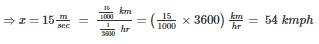QUESTION: 4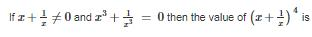Solution:

From the given data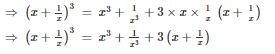But we know that x3 + 1/x3 = 0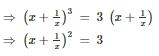Squaring on both sides, we get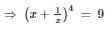QUESTION: 5

Trish and Stacy can complete a work in 20 days and 30 days, respectively, when working alone. In an arrangement, Trish works for 2 days, and then Stacy works for a day. They keep on repeating this process till the work gets completed. On which day will the work gets completed?​

Solution:

In given arrangement, Trish works for 2 days, and then Stacy works for a day. This cycle repeats till work is completed.
Fraction of work done by Trish and Stacy in a day is 1/20 and 1/30, respectively.
Work completed in 1 cycle of 3 days = 2/20 + 1/30 = 4/30
⇒ Work completed in 7 cycles of 3 days = 28/30
Work done till first day of 8th cycle = 28/30 + 1/20 = 59/60
Work done till second day of 8th cycle = 59/60 + 1/20 = 62/60
⇒ Work gets completed on 2nd day of 8th cycle, i.e., on 23rd day.

QUESTION: 6

If the sum of the measures of all the interior angles of polygon is 1080°, find the number of sides of the polygon?

Solution:

We know that sum of the interior angles of a polygon of n sides = 180 (n - 2)
Given that sum of the measures of all the interior angles = 1080°
⇒ 180(n – 2) = 1080
⇒ n – 2 = 6
⇒ n = 8

QUESTION: 7

If 2cosec2A = x, then find the value of x?

Solution:

From given data, we get
⇒ 2cosec2 A = x
⇒ 2/sin2 A = x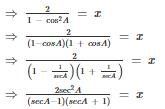QUESTION: 8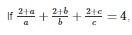then the value of (ab + bc + ca)/abc is

Solution: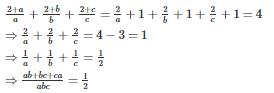QUESTION: 9

In an examination, 35% of total students failed in Hindi, 45% failed in English and 20% failed in both. Find the percentage of those students who passed in both the subjects?

Solution:

From given data
No of students failed in Hindi n(H) = 35%
No of students failed in English n(E) = 45%
No of students failed in both n(H ∩ E) = 20%
⇒ total number of students who failed n(H ⋃ E) = n(H) + n(E) – n(H ∩ E)
⇒ n(H ⋃ E) = 35 + 45 - 20
⇒ n(H ⋃ E) = 60
No of students who passed = 100 - (total no of students who failed) = 100 – 60 = 40%

QUESTION: 10

A sum of Rs. 3000 amounts to Rs. 6000 in two years at compound interest. The interest for four years is

Solution:

From the given data
We know amount = p(1 + r/100)n
⇒ 3000(1 + r/100)2 = 6000
⇒ (1 + r/100)2 = 2
Compound interest for four years = amount – principle
= p(1 + r/100)n – principle
= 3000(1 + r/100)– 3000 = 12000 – 3000 = Rs. 9000

QUESTION: 11

If the diagonals of a rhombus are 24 and 10, then the value of thrice its side is

Solution:

Half of each of the two diagonal forms the two sides of a right - angled triangle whose hypotenuse is the side of the rhombus.
Length of Diagonal 1 = 24
Length of Diagonal 2 = 10
⇒ Sides of the triangle = 12 and 5

Since it’s a right - angled triangle, it should follow Pythagoras theorem.
⇒ (Side)2 = (12)2 + (5)2
⇒ Side = 13
∴ Side of the rhombus = 13.
⇒ Thrice the value of the side = 3 × 13 = 39

QUESTION: 12

If the measures of the angles of a triangle are in the ratio. 1 ∶ 2 ∶ 3 and if the length of the smallest side of the triangle is 10 cm, then the length of the longest side is

Solution:

Let the angles of the triangles are in the ratio x ∶ 2x ∶ 3x
We know that sum of the angles in a triangle = 180°
⇒ x + 2x + 3x = 180
⇒ 6x = 180°
⇒ x = 30°
by sin formula sinA/a = sinB/b = sinC/c
∴ the angles of the triangle are 30°, 60° and 90°, so the sides are in the ratio of 1 ∶ 2 ∶ √3
∴ smallest side will be opposite to 30°
Let the sides of the triangle are in the ratio of x : 2x : √3x
But given smallest side x = 10 cm
⇒ remaining sides are 2x = 2(10) = 20 cm and √3x = √3(10) = 17.32 cm
∴ the longest side = 20 cm

QUESTION: 13

Two numbers are respectively 30% and 80% more than a third number. Find the ratio of these numbers.

Solution:

Let the third number be X.
Let the first number be A, and the other number be B. We have,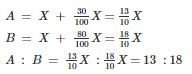QUESTION: 14

A cricketer has a certain average of runs for his 6 innings. In the seventh inning he scores 100 runs, thereby increasing his average by 7 runs. His new average of runs is

Solution:

Let the average of 6 innings be x.
Then new average can be found by (6x + 100)/7 = x + 7
⇒ 6x + 100 = 7x + 49
⇒ x = 51
∴ New average = 51 + 7 = 58

QUESTION: 15

If a3 ­ - b3 = 56 and a ­ - b = 2 then what is the value of a2 + b2?​

Solution:

a– b3 = (a – b)(a2 + ab + b2)
56 = 2(a2 + ab + b2)
28 = (a2 + ab + b2) ----(1)
Now, (a – b)2 = a2 – 2ab + b2 = 4 ----(2)
Solving equation (1) and (2),
a2 + ab + b2 = 28
a2 – 2ab + b2 = 4
3ab = 24
ab = 8
Therefore, a2 + 8 + b2 = 28 in (1)
And a2 + b2 = 28 – 8 = 20

QUESTION: 16

In ΔABC, ∠B = 5∠C and ∠A = 3∠C, then the measure of ∠C is

Solution:

We know that in a triangle,
Sum of angles = 180°
⇒ ∠A + ∠B + ∠C = 180°
⇒ 3∠C + 5∠C + ∠C = 180°
⇒ 9∠C = 180°
⇒ ∠C = 180/9 = 20°
∴ ∠C = 20°

QUESTION: 17

A pole stands vertically, inside a scalene triangular park ABC. If the angle of elevation of top of the pole from each corner of the park is same, then in Δ ABC, the foot of the pole is at the

Solution:

tanθ = perpendicular/base
Given, a pole stands vertically, inside a scalene triangular park ABC. The angle of elevation of top of the pole from each corner of the park is same.
If the angle of elevation is same then, the distance of each corner from the foot of the would also be same,
Base = perpendicular/tanθ
Perpendicular is height of the pole while θ is the angle of elevation.
For the above to be true, the foot of the pole should be a circumcenter and the distance of each corner from the foot is the circumradius.

QUESTION: 18

Three sides of a triangle are 5 cm, 9 cm and x cm. The minimum integral value of x is

Solution:

From the given data
Length of sides of a triangle are of 5 cm, 9 cm and x cm
⇒ length of the third side must be less than the sum of the other two sides
⇒ x < 5 + 9 or x < 14
We also know that length of the third side must be greater than difference of the other two sides
⇒ x > 9 – 5 or x > 4
⇒ By both the conditions 4 < x < 14

QUESTION: 19

The length of a chord which is at a distance of 5cm from the centre of a circle of radius 13cm is

Solution:

From given data
Let AB be a chord of circle with center O and radius OA and OB equals to 13 cm
Draw OP perpendicular to AB
Given that OP = 5 cm
From ΔOPA, we know that
⇒ OA2 = AP2 + OP2
⇒ 132 = AP2 + 52
⇒ 169 – 25 = AP2
⇒ AP = 12 cm
But we know that AB = 2 AP = 2× 12 = 24 cm

QUESTION: 20

Divide 43 into two parts so that 4 times the first and 3 times the second together equals to 150. Then ratio of the first and second parts is

Solution:

Let the first part of 43 be x, then the second part will be 43 – x
According to the question,
4x + 3 × (43 – x) = 150
⇒ 4x + 129 – 3 x=150
⇒ x = 21
∴ The first part is 21 and second part is 43 – 21 = 22

QUESTION: 21

25% discount is already offered on an item. By applying a promo code the customer wins 20% cash back. What is the effective discount?

Solution:

Let the cost price of the item be Rs. 100
25% discount is offered on an item
⇒ discount = 100 × 25/100 = Rs. 25
∴ discounted selling price = 100 - 25 = Rs. 75
Again 20% cash back is offered
⇒ cash back amount = 75 × 20/100 = Rs. 15
∴ final selling price = 75 - 15 = Rs. 60
Effective discount = cost price – selling price/cost price × 100 = 100 - 60/100 × 100 = 40%

QUESTION: 22

Refer the below data table and answer the following Question :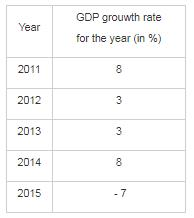If the GDP of the country was \$3 trillion at the end of 2013, what was it at the beginning of 2015?​

Solution:

As per the given data,
3\$ was the GDP at the end of 2013.
It was given that to find at the beginning of 2015, it means to find the GDP at the end of 2014. At the end of 2014, there was the increase of GDP to 8%.
⇒ total amount at the end of 2014 = 3 trillion + (8% of 3 trillion) = 3.24 trillion
∴ GDP at the beginning of 2015 was \$3.24 trillion.

QUESTION: 23

Refer the below data table and answer the following Question :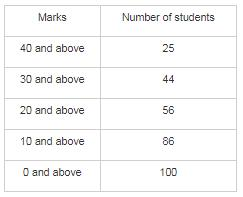Q. Find the number of students whose marks are between 30 and 40 ?

Solution:

As per the given data
Similarly no of students who got marks 30 and above = 44
Similarly no of students who got marks 40 and above = 25
∴ no of students whose marks are between 30 and 40 = 44 – 25 = 19

QUESTION: 24

Direction: Study the following graph and answer the question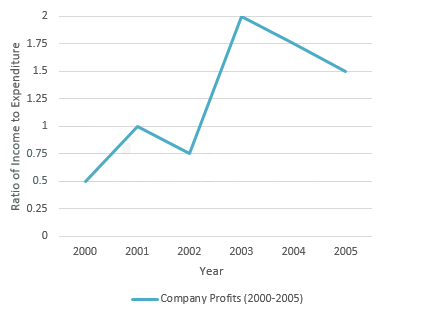Q. If the profit made in the year 2003 was Rs. 450000, then find the income

Solution:

From the line graph,
Ratio of income to expenditure in the 2003 = 2
Let the expenditure be a, ∴ income = 2a
Profit = income – expenditure
⇒ 450000 = 2a – a
⇒ a = Rs. 450000
Thus, income = 2a = Rs. 900000

QUESTION: 25

Direction: Study the following graph and answer the questionQ. Find the percentage increase in income from 2002 to 2003​

Solution:

The line graph is drawn between ratio of income to expenditure and annual year.
We don’t know the income in each year.
Thus, the data given is inadequate.